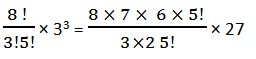Study Materials: ncert solutions

Our ncert solutions for Exercise 8.2 Class 11 maths 8. Binomial Theorem - ncert solutions - Toppers Study is the best material for English Medium students cbse board and other state boards students.

Solutions ⇒ Class 11th ⇒ Mathematics ⇒ 8. Binomial Theorem

# Exercise 8.2 Class 11 maths 8. Binomial Theorem - ncert solutions - Toppers Study

Topper Study classes prepares ncert solutions on practical base problems and comes out with the best result that helps the students and teachers as well as tutors and so many ecademic coaching classes that they need in practical life. Our ncert solutions for Exercise 8.2 Class 11 maths 8. Binomial Theorem - ncert solutions - Toppers Study is the best material for English Medium students cbse board and other state boards students.

## Exercise 8.2 Class 11 maths 8. Binomial Theorem - ncert solutions - Toppers Study

CBSE board students who preparing for class 11 ncert solutions maths and Mathematics solved exercise chapter 8. Binomial Theorem available and this helps in upcoming exams 2023-2024.

### You can Find Mathematics solution Class 11 Chapter 8. Binomial Theorem

• All Chapter review quick revision notes for chapter 8. Binomial Theorem Class 11
• NCERT Solutions And Textual questions Answers Class 11 Mathematics
• Extra NCERT Book questions Answers Class 11 Mathematics
• Importatnt key points with additional Assignment and questions bank solved.

NCERT Solutions do not only help you to cover your syllabus but also will give to textual support in exams 2023-2024 to complete exercise 8.2 maths class 11 chapter 8 in english medium. So revise and practice these all cbse study materials like class 11 maths chapter 8.2 in english ncert book. Also ensure to repractice all syllabus within time or before board exams for ncert class 11 maths ex 8.2 in english.

See all solutions for class 11 maths chapter 8 exercise 8 in english medium solved questions with answers.

### Exercise 8.2 class 11 Mathematics Chapter 8. Binomial Theorem

• Exercise 8.2 Class 11 Maths 8. Binomial Theorem - Ncert Solutions - Toppers Study
• Class 11 Ncert Solutions
• Solution Chapter 8. Binomial Theorem Class 11
• Solutions Class 11
• Chapter 8. Binomial Theorem Exercise 8.2 Class 11

## Exercise 8.2 Class 11 maths 8. Binomial Theorem - ncert solutions - Toppers Study

Exercise 8.2

Find the coefficient of

Q1.  x5 in (x + 3)8

Solution:

(x + 3)8

General term nCr an-r br

n = 8, then

8Cr x8-r 3r x5 = x8-r

5 = 8-r

∴ r = 8-5 = 3

8C3 x8-3 33 = 8C3 x5 33

Coefficient in x5 = 8C3 33= 1512

Q2.  a5b7 in (a-2b)12

Solution:

(a-2b)12

General Term Tr+1 = nCr an-r br

12Cr a12-r br

12 – r = 5  r = 12 – 5 = 7

a5b7 is 8 term

T8 = 12C7 a5 b7

= 12C7 a5 (-2b)7= - 72 × 11 × 27

= - 72 × 11 × 128 = - 101376

Write the general term in the expansion of

Q3. (x2 - y)6

Solution:

General term of (x2 - y)6

n = 6, a = x2, b = (-y)

General Term Tr +1nCan-r br

=  6Cr (x2)6-r (-y)r

##### Other Pages of this Chapter: 8. Binomial Theorem

Important Study materials for classes 06, 07, 08,09,10, 11 and 12. Like CBSE Notes, Notes for Science, Notes for maths, Notes for Social Science, Notes for Accountancy, Notes for Economics, Notes for political Science, Noes for History, Notes For Bussiness Study, Physical Educations, Sample Papers, Test Papers, Mock Test Papers, Support Materials and Books.Mathematics Class - 11th

NCERT Maths book for CBSE Students.

books

## Study Materials List:

##### Solutions ⇒ Class 11th ⇒ Mathematics
1. Sets
2. Relations and Functions
3. Trigonometric Functions
4. Principle Of Mathematical Induction
5. Complex Numbers and Quadratic Equations
6. Linear Inequalities
7. Permutations and Combinations
8. Binomial Theorem
9. Sequences and Series
10. Straight Lines
11. Conic Sections
12. Introduction to Three Dimensional Geometry
13. Limits and Derivatives
14. Mathematical Reasoning
15. Statistics
16. Probability

New Books